# Relationship between Angular momentum and torque of the system of particles

## Relationship between Angular momentum and torque of the system of particles

(A) Angular momentum of system of particles
• Consider a system of particles made up of number of particles, moving independently to each other. Let L1, L2,L3,.............etc. be the angular momentum of different particles of the system w.r.t., to a given point.
• The angular momentum of the particle system w.r.t. that given point is equal to the vector sum of angular momentum of all the particles of the system.
• If L is the angular momentum of the system of particles or the body as a whole then,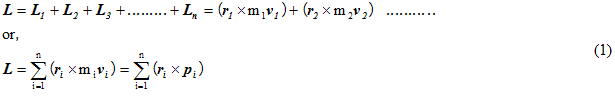(B) Torque acting on system of particles
• Torque acting on the system of particles from equation above angular momentum of the system of particles is given as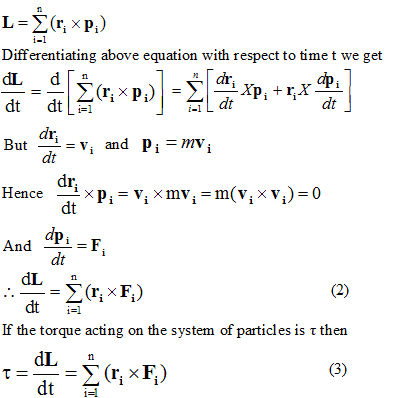• When particles of the system are in motion then their motion is due to external and interaction due to internal forces so force acting on any particle of the system is given by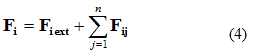Here Fiext is the external force acting on the ith particle and ∑Fij is the sum of the force acting on the particle due to internal interaction of different particles.Putting the value of Fi in the equation we get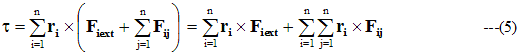• RHS of the equation 2 shows that summation of the moment of interacting force (internal) .Here internal interaction forces balance each other so torque due to internal forces adds to zero hence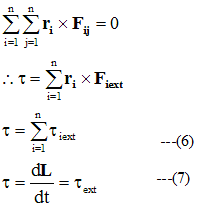• Above equation proves that total torque acting on a system of particles is equal to the vector sum of the torque acting on the different particles due to external force on the particle and its value is also equal to the rate of change of angular momentum

## Angular momentum of the system of particles with respect to the center of mass of the system

• let a system of particles is made up of n number of particles.Let ri be the position vector of the ith particle P with respect to a point O and vi be its velocity .let Rcm be the position vector of Center of mass C of the system with respect to the origin
• Let ri' and vi' be the position vector and velocity vector of the ith particle with respect to center of mass of the system.
• Angular momentum of the system of particles with respect to origin is given by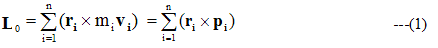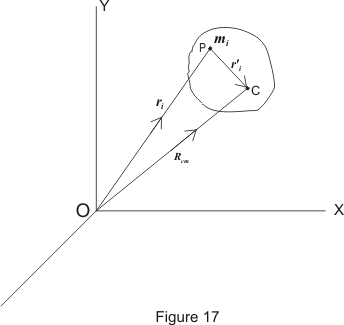• Angular momentum of the system of particles with respect to center of mass of the system is given by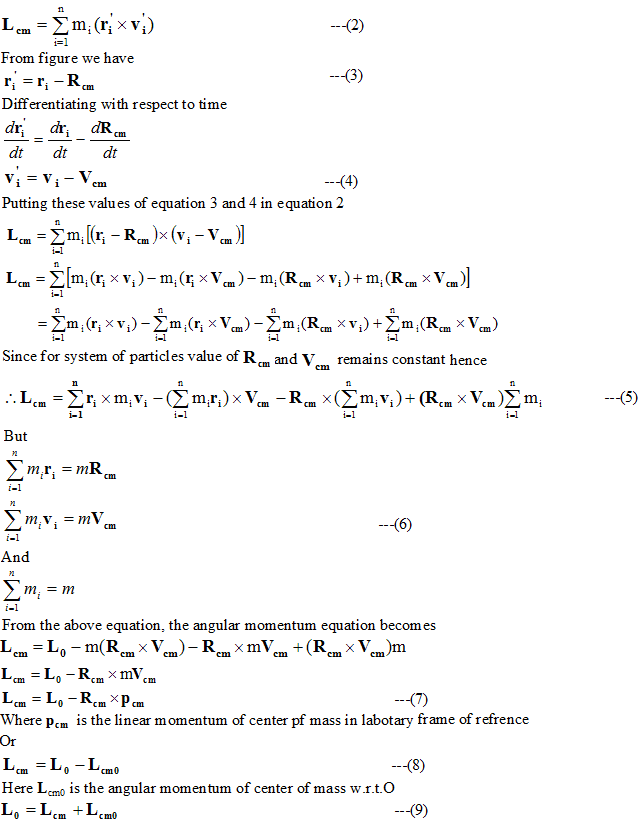Hence the angular momentum of the system of the particles with respect to point O is equal to the sum of the angular momentum of the center of mass of the particles about O and angular momentum of the system about center of mass